# Three Hidden Rectangles

Alignments to Content Standards: 3.MD.C.7.d

There are many ways to find the area of this figure.1. Try to find as many ways as you can to split this figure into exactly 3 rectangles. Be sure that none of the rectangles overlap and the 3 rectangles cover the entire figure.
2. For every example you found in part a, write an expression that represents the area as the sum of the three rectangles.
3. Find the total area of this figure.

## IM Commentary

The purpose of this task is for students to decompose a figure into rectangles and then find the total area by adding the area of all of its smaller, non-overlapping rectangles. This task also requires students to create expressions to represent the area of the entire figure as the sum of the areas of the rectangles. Students will need to see an example at the beginning, since there are different expressions that could be appropriate, and writing the directions unambiguously would be challenging reading for third graders.

This is an instructional task. The beginning is simple and allows… even invites… students to try things out that don’t work. Students should have multiple copies of the figure or access to graph paper so they can experiment. There will be rich opportunities to discuss whether students have satisfied the requirements. The second part of the task is more technical and can be used as a formative assessment to see whether students can create expressions to match their ideas.

This task relies heavily on the work students have done transitioning from array to area representations for multiplication. This task requires students to attend to precision (MP 6) as they must attend to the exact number of rectangles needed, ensure that the rectangles don’t overlap, and that the rectangles together cover the entire figure. Implicit in this is also that students understand how to identify whether their shapes are indeed rectangles.

Coaches and facilitators of professional development may also want to consider using this task, or similar tasks, in workshops for teachers. Many adults do not see the five possibilities right away. These tasks are great reminders to adults that they can be learners of mathematics, too!

## Solution

a. and b. There are 5 ways to create 3 non-overlapping rectangles that partition the figure: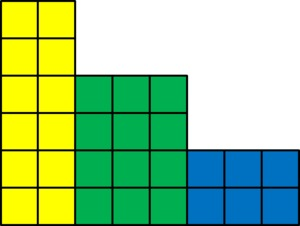Expression: 2×6+3×4+3×2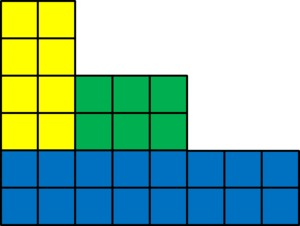Expression: 2×4+3×2+8×2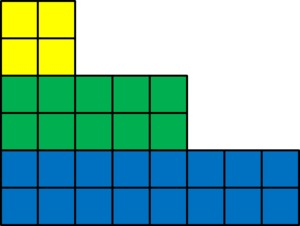Expression: 2×2+5×2+8×2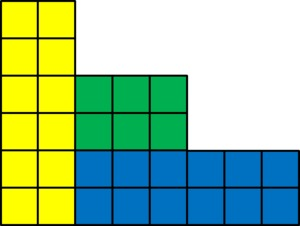Expression: 2×6+3×2+6×2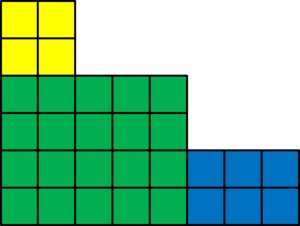Expression: 2×2+5×4+3×2

c. The total area of this figure is 30 square units. Each of these different pictures shows a different way to decompose this shape into three rectangles. In each of these five decompositions, the area of the three rectangles sums to 30 square units.